Get instant live expert help with Excel or Google Sheets“My Excelchat expert helped me in less than 20 minutes, saving me what would have been 5 hours of work!”

#### Post your problem and you'll get expert help in seconds

Your message must be at least 40 characters
Our professional experts are available now. Your privacy is guaranteed.

# How to Check If Range Contains a Value Not in Another Range in Excel

We often compare one range values with another range to check for duplicates. But how to check if one range values exist in another range in Excel? We will learn in this article how to check if a range contains a value not in another range in Excel.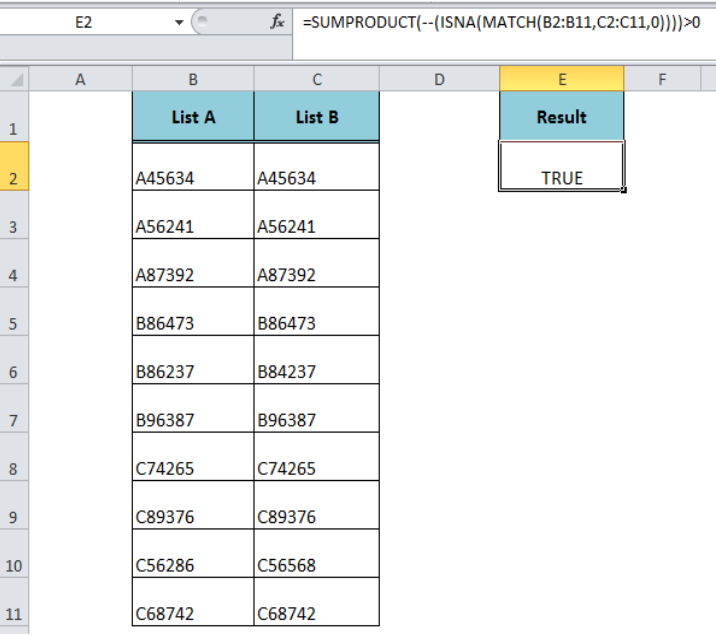Figure 1. Check If a Range Contains a Value Not in Another Range

## Formula Syntax

The generic formula syntax is;

`=SUMPRODUCT(--(ISNA(MATCH(range1,range2,0))))>0`

In this formula, we use the SUMPRODUCT function along with MATCH and ISNA function. This formula checks if range one contains at least one or more values that are not part of another range and returns TRUE, else it returns FALSE.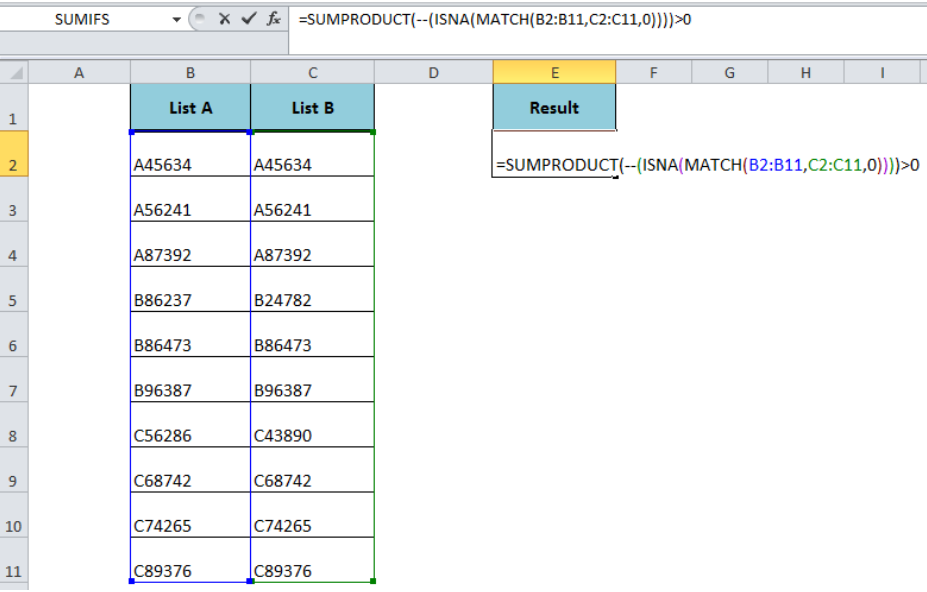Figure 2. Formula Syntax

Suppose we have two lists of students’ roll numbers and we want to check if List A contains any values (one or more values) that are not in List B. Hence, the formula compares List A (range B2: B11) with List B (range C2: C11) and returns TRUE if List A contains at least one or more values not in List B, such as;

`=SUMPRODUCT(--(ISNA(MATCH(B2:B11,C2:C11,0))))>0`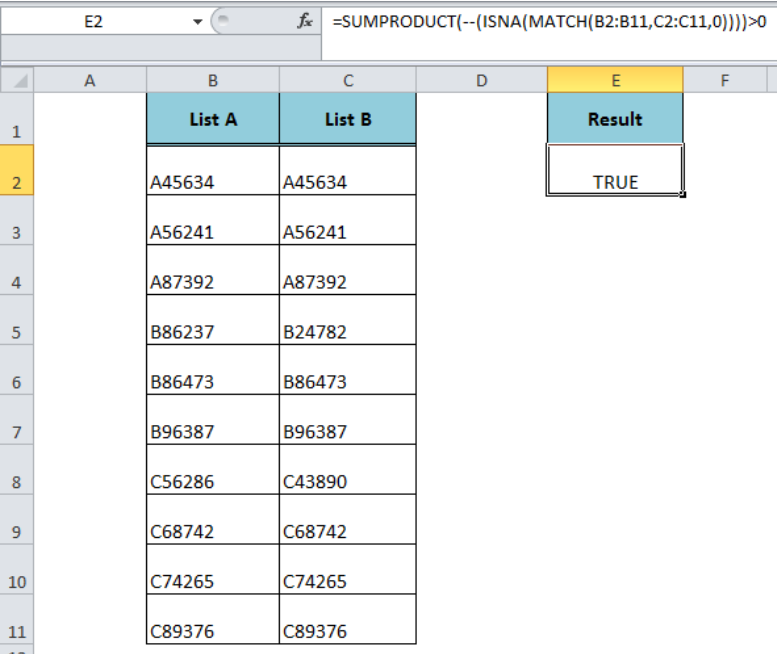Figure 3. The Formula Result

## How Formula Works

The MATCH function returns the relative positions of range B2: B11 values that exactly match in range C2: C11, and returns the #NA error value(s) where range B2: B11 values are not found in range C2: C11 as an array. Select the MATCH part of the formula in the formula bar and press F9 to view the resulting array.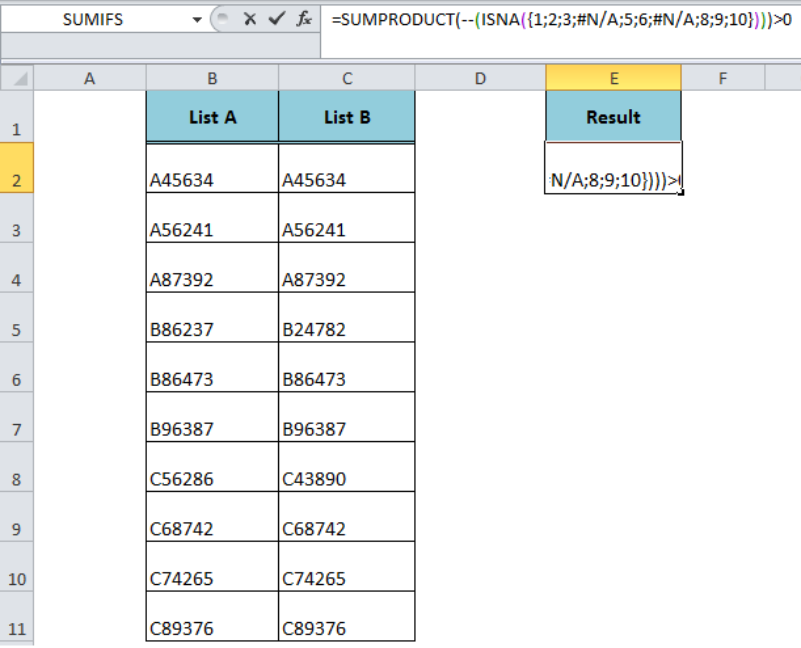Figure 4. Resulting Array of the MATCH Function

The ISNA function checks for the #NA error values and returns TRUE, else returns FALSE. Hence, it converts the array returned by the MATCH function into an array of TRUE and FALSE logical values, such as;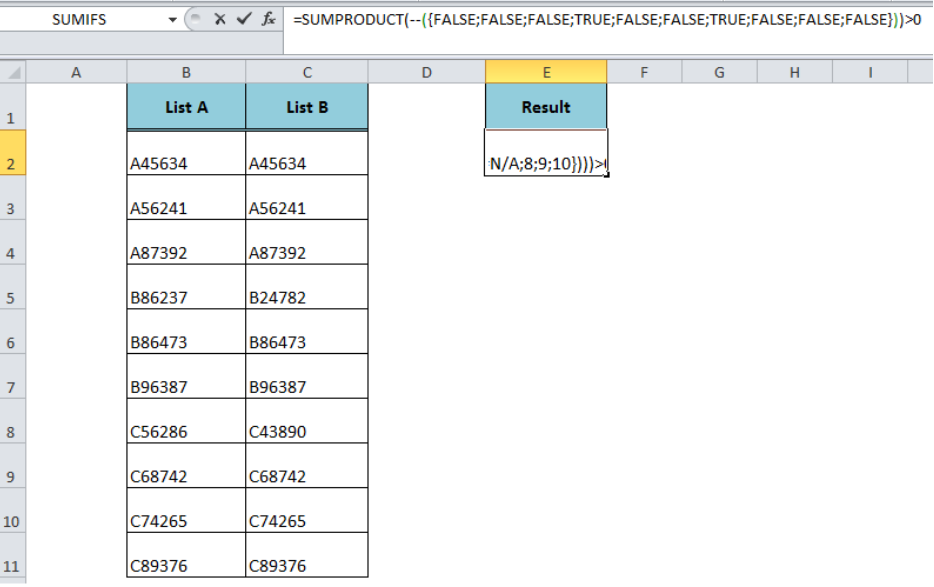Figure 5. The array of Logical Values Return by the ISNA Function

The double dash (–) symbol converts the TRUE and FALSE logical values into an array of 1s and 0s. The SUMPRODUCT function sums this final array of 1s and 0s and compares the result with 0 and returns TRUE if the sum of values is greater than 0.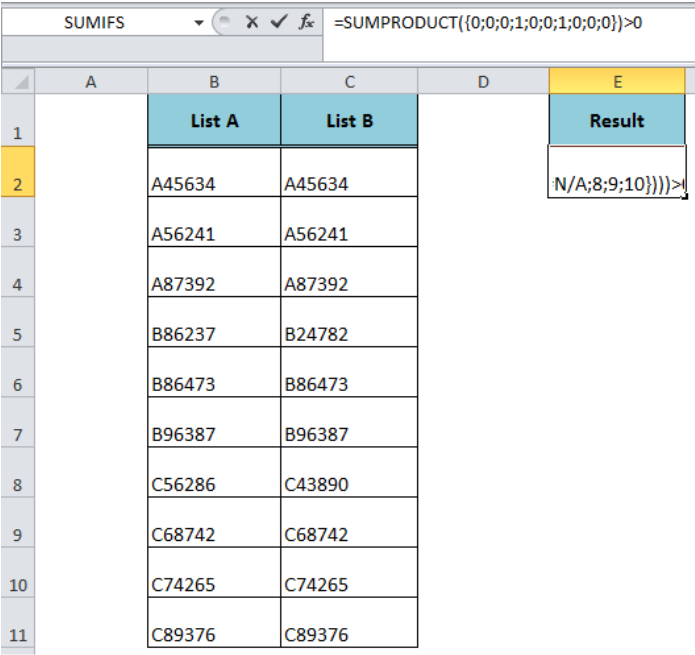Figure 6. Final Array of 1s and 0s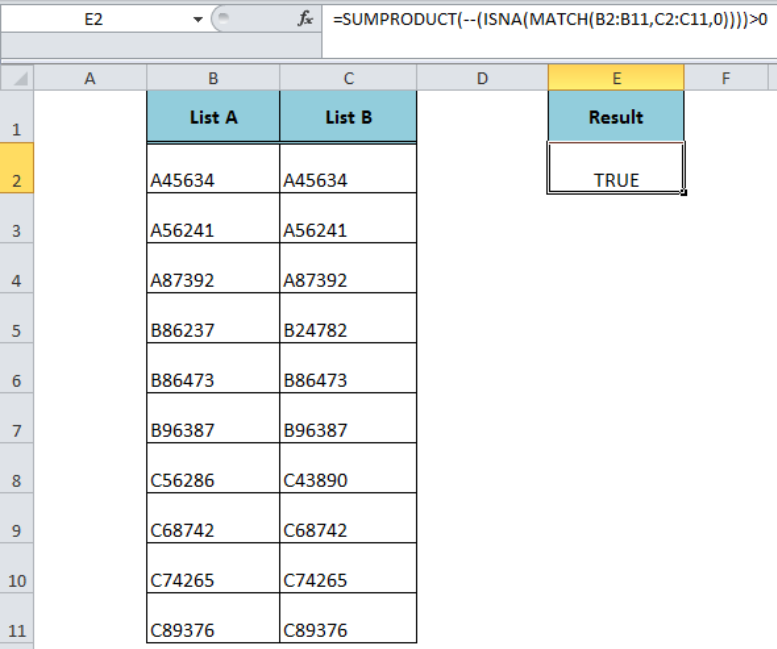Figure 7. Final Result of the Formula

## Instant Connection to an Expert through our Excelchat Service:

Most of the time, the problem you will need to solve will be more complex than a simple application of a formula or function. If you want to save hours of research and frustration, try our live Excelchat service! Our Excel Experts are available 24/7 to answer any Excel question you may have. We guarantee a connection within 30 seconds and a customized solution within 20 minutes.

### Did this post not answer your question? Get a solution from connecting with the expert.Another blog reader asked this question today on Excelchat:## Subscribe to Excelchat.coAnother blog reader asked this question today on Excelchat: